﻿ 基于奇异谱分析的CORS大地高时序形变信息提取文章快速检索 高级检索
 大地测量与地球动力学2019, Vol. 39Issue (8): 825-831  DOI: 10.14075/j.jgg.2019.08.012### 引用本文LI Wanqiu, WANG Wei, ZHANG Chuanyin, et al. Deformation Signal Extraction of Geodetic Height Change Time Series of CORS Based on Singular Spectrum Analysis[J]. Journal of Geodesy and Geodynamics, 2019, 39(8): 825-831.### Foundation support

National Natural Science Foundation of China, No. 41374081, 41674024; National Key Research and Development Program of China, No. 2016YFB0501702; Basic Research Fund of Chinese Academy of Surveying and Mapping, No.7771806;Special Fund for Basic Scientific Research of Central Public Research Institutes, No.AR1905.

### Corresponding author

WANG Wei, PhD,associate researcher, majors in gravity field and vertical reference,E-mail:wangwei@casm.ac.cn.

### 第一作者简介

LI Wanqiu, PhD candidate, majors in the application of deformation monitoring and timely variable gravity field ,E-mail: 1404855692@qq.com.

### 文章历史

1. 中国测绘科学研究院，北京市莲花池西路28号，100830;
2. 山东科技大学测绘科学与工程学院，青岛市前湾港路579号，266590;
3. 中国地质大学(武汉)信息工程学院，武汉市鲁磨路388号，43007;
4. 青岛市勘察测绘研究院，青岛市山东路189号，266032;
5. 云南大学国际河流与生态安全研究院，昆明市云学路，650500

CORS大地高时序中通常混有噪声误差，除了包含环境负荷及非负荷垂向形变外，还包含仪器误差造成的影响，抑制了形变信息的精度与可靠性，因此分离基准站的垂向形变信号与噪声，确定其可靠变化趋势尤为重要。消除时间序列中仪器噪声常用的方法有最小均方滤波和最小二乘滤波。最小均方滤波要求输入的序列具有平稳性，而最小二乘滤波则适用于非平稳序列，并已知序列中噪声的先验统计信息。另外，噪声误差中的高频部分亦可采用低通滤波方式消除，但该方法难以适用于全频段误差的改正。构建时间序列自协方差矩阵，根据其特征值变化反映有用信号与噪声的分布，可采用奇异谱分析方法实现CORS时序的降噪处理。

1 SSA方法原理

SSA是一种主成分分析的时序处理方法，针对中心化后的一维时间序列，按给定的嵌入空间维数构造时滞矩阵，并截断一定个数的主分量，重构趋势与周期项信号

 $\begin{array}{l} \mathit{\boldsymbol{X}} = \left[ {\begin{array}{*{20}{c}} {{x_1}}&{{x_2}}& \cdots &{{x_{i + 1}}}& \cdots &{{x_{N - M + 1}}}\\ {{x_2}}&{{x_3}}& \cdots &{{x_{i + 2}}}& \cdots &{{x_{N - M + 2}}}\\ \vdots & \vdots & \vdots & \vdots & \vdots & \vdots \\ {{x_M}}&{{x_{1 + M}}}& \cdots &{{x_{i + M}}}& \cdots &{{x_N}} \end{array}} \right] = \\ \;\;\;\;\;\;\left[ {\begin{array}{*{20}{c}} {{X_1}}&{{X_2}}& \cdots &{{X_{i + 1}}}& \cdots &{{X_{N - M + 1}}} \end{array}} \right] \end{array}$ (1)

1) 计算空间坐标矩阵XM×N维自协方差矩阵Tx及其特征值λk与特征向量Ek

 $\begin{array}{*{20}{c}} {{\mathit{\boldsymbol{T}}_x} = }\\ {\left[ {\begin{array}{*{20}{c}} {c\left( 0 \right)}&{c\left( 1 \right)}& \cdots & \cdots & \cdots & \cdots &{c\left( {M - 1} \right)}\\ {c\left( 1 \right)}&{c\left( 0 \right)}&{c\left( 1 \right)}& \cdots & \cdots & \cdots & \cdots \\ \cdots &{c\left( 1 \right)}& \cdots & \cdots & \cdots & \cdots & \cdots \\ \vdots & \vdots & \vdots & \vdots & \vdots & \vdots & \vdots \\ \cdots & \cdots & \cdots & \cdots & \cdots & \cdots &{c\left( 1 \right)}\\ {c\left( {M - 1} \right)}& \cdots & \cdots & \cdots & \cdots &{c\left( 1 \right)}&{c\left( 0 \right)} \end{array}} \right]} \end{array}$ (2)

 $c\left( j \right) = \frac{1}{{N - j}}\sum\limits_{t = 1}^{N - j} {{x_t}{x_{t + j}}} , 0 \le j \le M - 1$ (3)

2) 计算向量XiEk上的投影。时序的k个主成分(PC)aik可表示为第i个状态向量XiEk上的投影，即

 $\begin{array}{l} \;\;\;\;\;\;\;\;\mathit{\boldsymbol{a}}_i^k = {\mathit{\boldsymbol{X}}_i}{\mathit{\boldsymbol{E}}^k} = \\ \sum\limits_{j = 1}^M {{x_{i + j}}\mathit{\boldsymbol{E}}_j^k} {\rm{ }}, {\rm{ }}0 \le i \le M - 1 \end{array}$ (4)

3) 重建成分序列。根据投影aik与特征向量Ek可得到各个主成分重构序列(RC)：

 $\ \ \ \ \ \ \ \ \ \ \ \ \ \ \ \ \ \ \ \ \ \ \ \ \ \ \ \ \mathit{\boldsymbol{x}}_i^k=\\ \left\{ \begin{array}{l} \frac{1}{i}\sum\limits_{j = 1}^M {\mathit{\boldsymbol{a}}_{i - j}^k\mathit{\boldsymbol{E}}_j^k, 1 \le i \le M - 1} \\ \frac{1}{M}\sum\limits_{j = 1}^M {\mathit{\boldsymbol{a}}_{i - j}^k\mathit{\boldsymbol{E}}_j^k, \mathit{M} \le i \le N - M + 1} \\ \frac{1}{{N - i + 1}}\sum\limits_{j = i - N + M}^M {\mathit{\boldsymbol{a}}_{i - j}^k\mathit{\boldsymbol{E}}_j^k, N - M + 2 \le i \le N} \end{array} \right.$ (5)

4) 重构时间序列。PC能够反映时间序列的变化特征，可根据能量相对关系分离时序形变信号与噪声，截断合适个数的主成分分量有利于形变的提取。为提高信噪比，本文截取顺序排列中贡献大的PC进行时序重构，降低噪声影响：

 $\mathit{\boldsymbol{\hat x}}{_i} = \sum\limits_{k = 1}^p {\mathit{\boldsymbol{x}}_i^k} , i = 1, 2, \cdots , N$ (6)
2 算例分析图 1 CORS位置分布 Fig. 1 The map of CORS position

CORS实测数据中混入粗差会影响空间坐标矩阵的自协方差矩阵构建，并且根据误差传播定律可知，还会导致自协方差矩阵特征值与特征向量求解产生偏差，影响主成分分量重构与噪声分离的精度。因此，准确定位并剔除时间序列中的粗差是序列分析的基础。对于时间序列粗差剔除的方法有多种，包括拉依达准则和IQR(四分位距)准则，由于时序本身粗差对中位数和IQR影响较小，相比拉依达准则，IQR准则更具稳健性。除了粗差影响之外，数据还存在由测量仪器等导致的缺数情况，也存在由于受地震、接收机或天线变动等影响导致的数据阶跃。当数据存在明显的阶跃时，应首先对其进行阶跃项改正，然后进行缺失数据补偿。

1) 阶跃项改正。采用最小二乘线性拟合方法消除阶跃项，利用阶跃发生前后时间段内时间序列拟合直线在阶跃点处的截距差值，分析和修复线性趋势改变对时间序列的影响。图 2为GSTS站点U分量阶跃项经最小二乘拟合修复后的结果。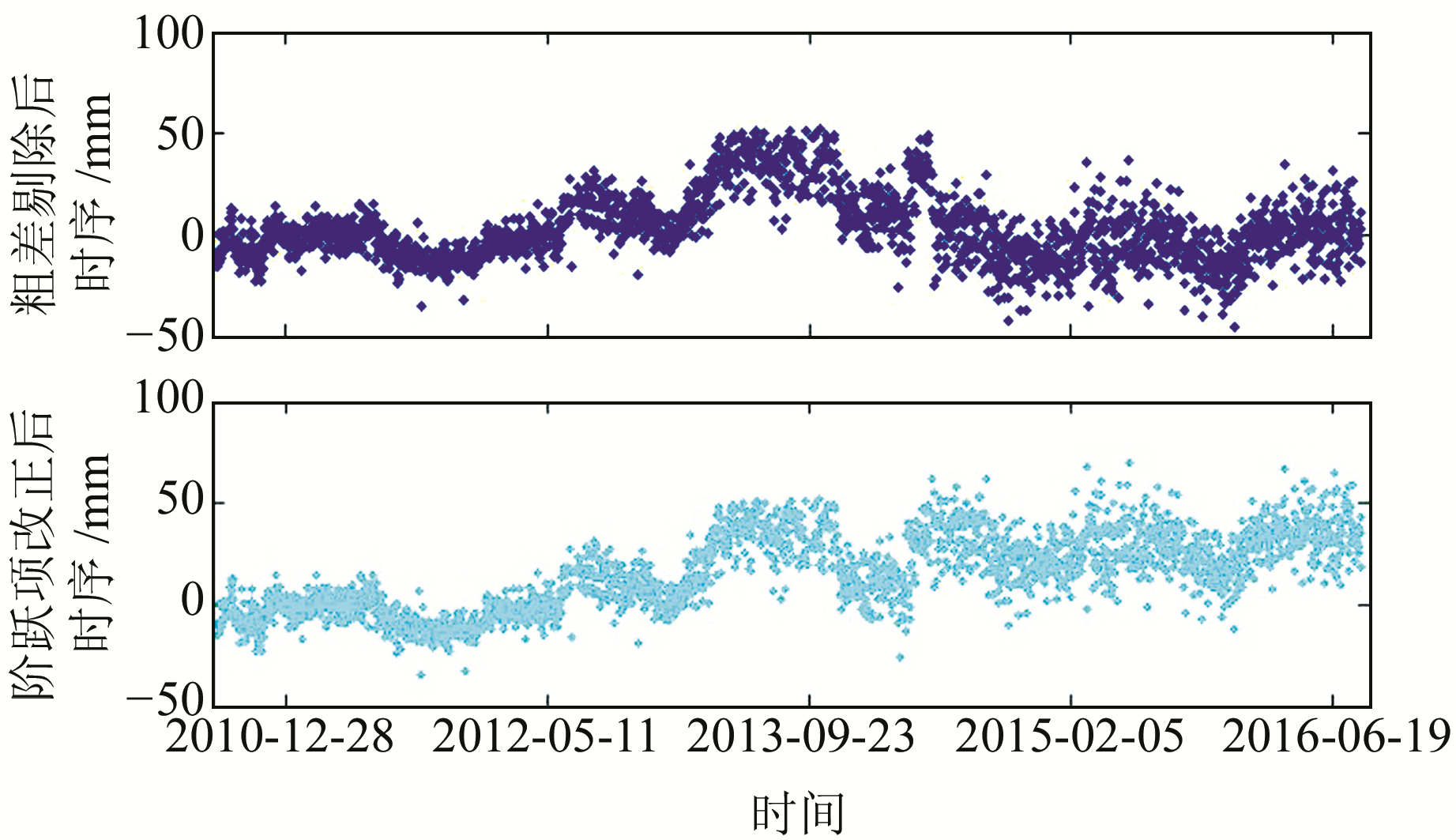图 2 粗差剔除及阶跃项改正后的GSTS站大地高变化时序 Fig. 2 Geodetic height change time series from GSTS station after gross error elimination and step correction

2) 缺失数据补偿。对序列基于最小二乘原理拟合其常数项、线性项、1 a项及0.5 a项，对缺失时段补偿拟合时序，并添加相应的噪声，添加的噪声序列默认为理想白噪声。图 3为SNYA站点U分量观测数据经缺失补偿后的结果。图 3 粗差剔除及缺失数据补偿后的SNYA站大地高变化时序 Fig. 3 Geodetic height change time series from SNYA station after gross error elimination and missing data compensation

2.1 仿真及结果

 $H\left( t \right) = {H_0}\left( t \right) + n\left( t \right)$ (7)
 ${H_0}\left( t \right) = 10{\rm{sin}}(\frac{{2\pi }}{{365}}t)$ (8)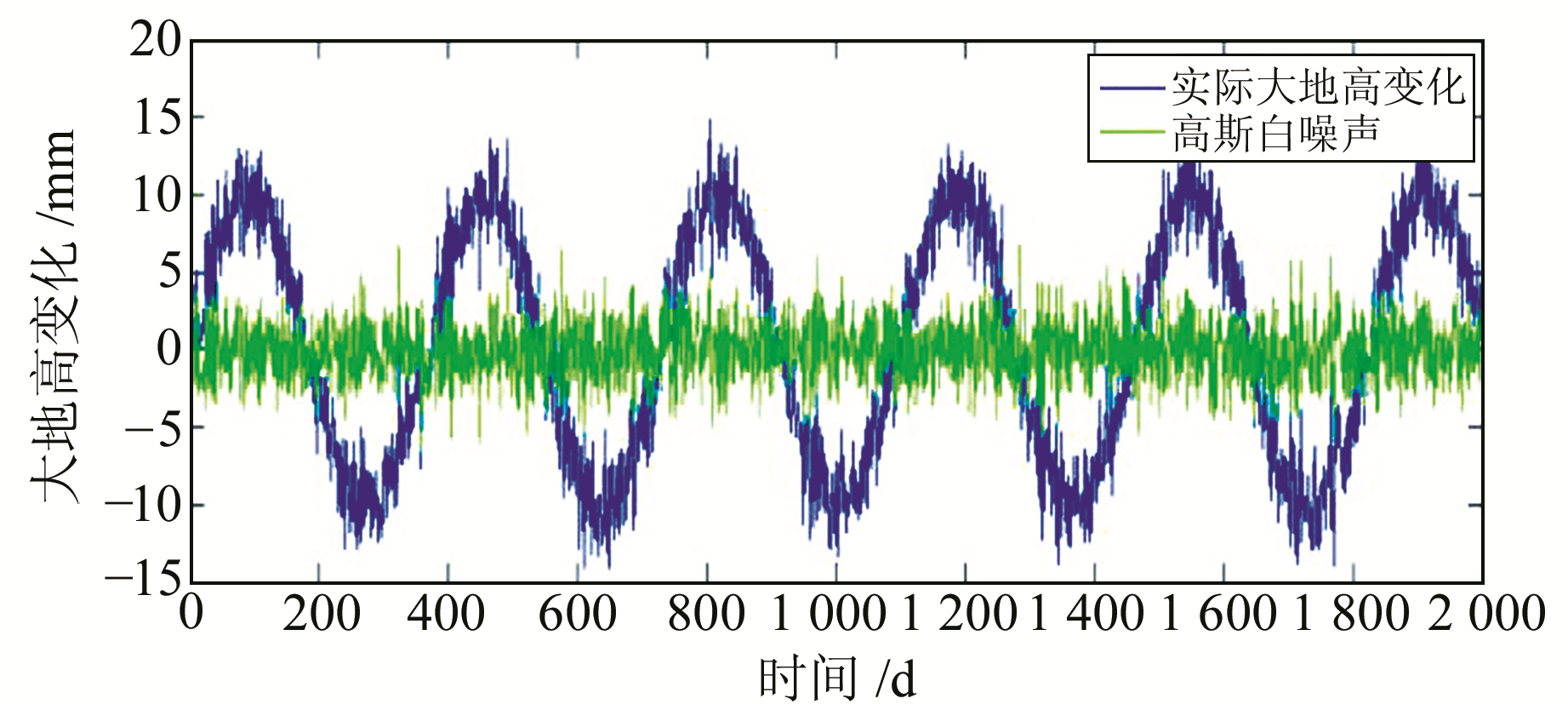图 4 模拟的CORS大地高变化实际时序与高斯白噪声 Fig. 4 Simulated actual time series of geodetic height change from CORS and Gaussian white noise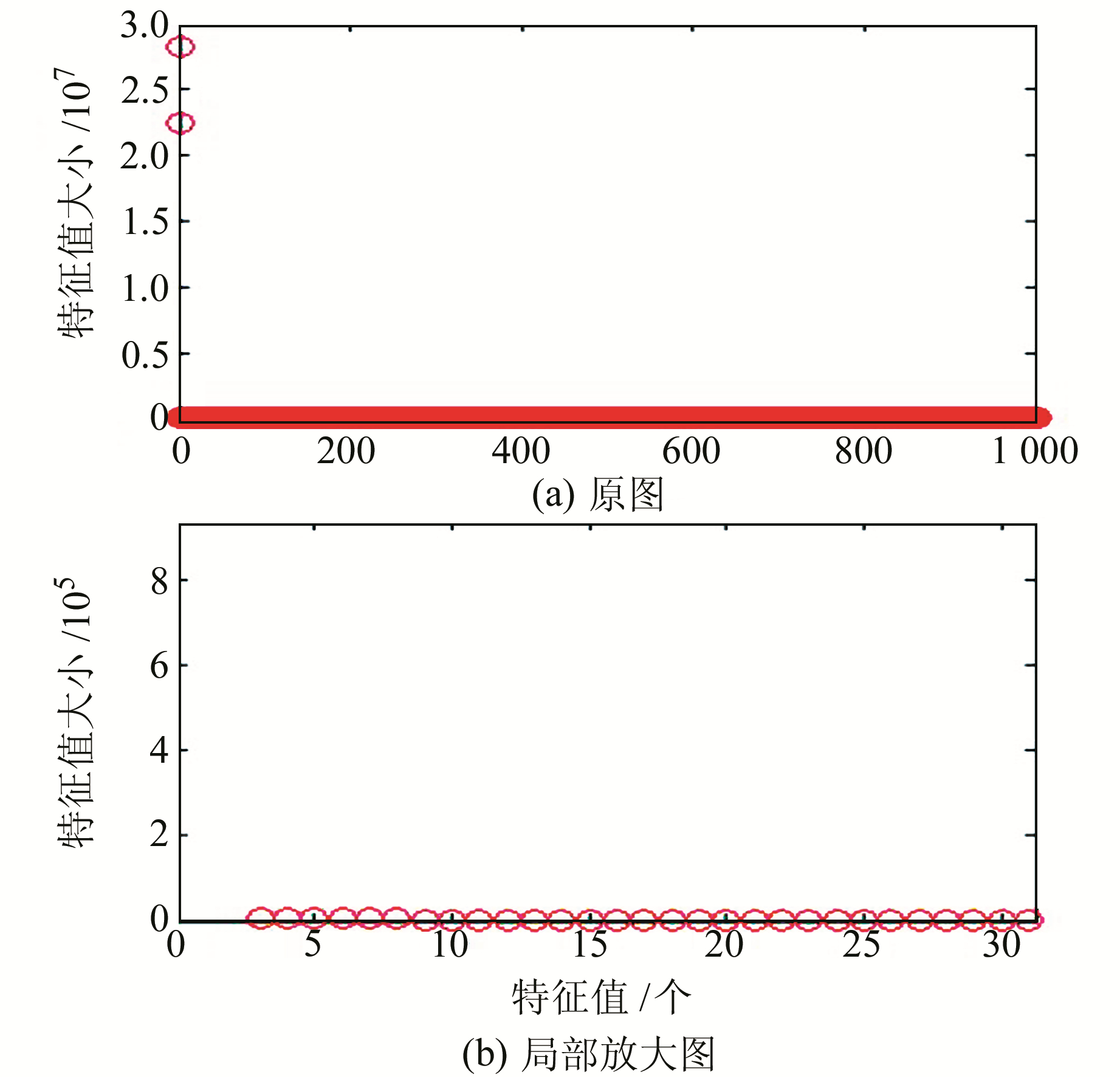图 5 模拟的CORS大地高变化时序特征值变化曲线 Fig. 5 Simulated eigenvalue change curve based on geodetic height change time series from CORS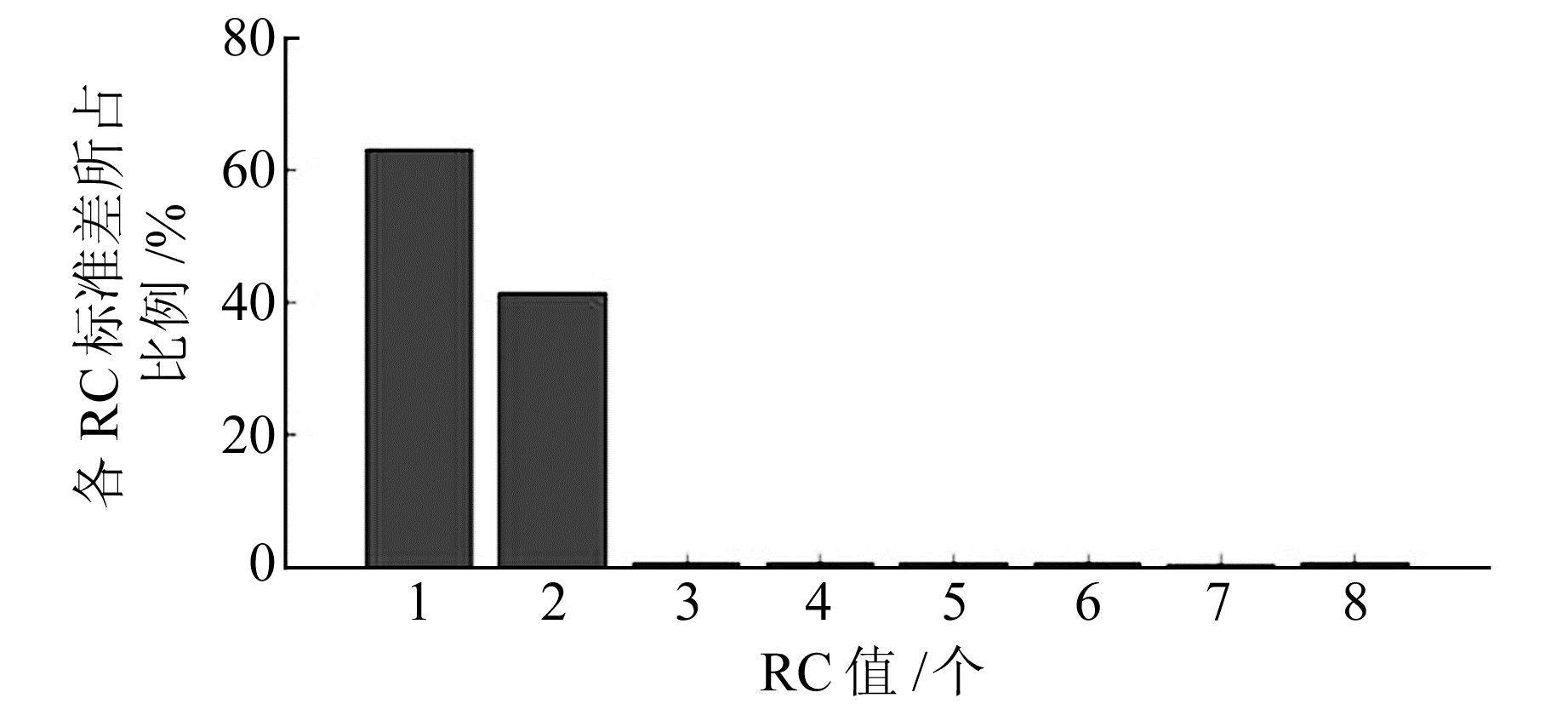图 6 前8阶各RC标准差占原始时序标准差的百分比 Fig. 6 The first 8 stages of each RC standard deviation as a percentage of the original time standard deviation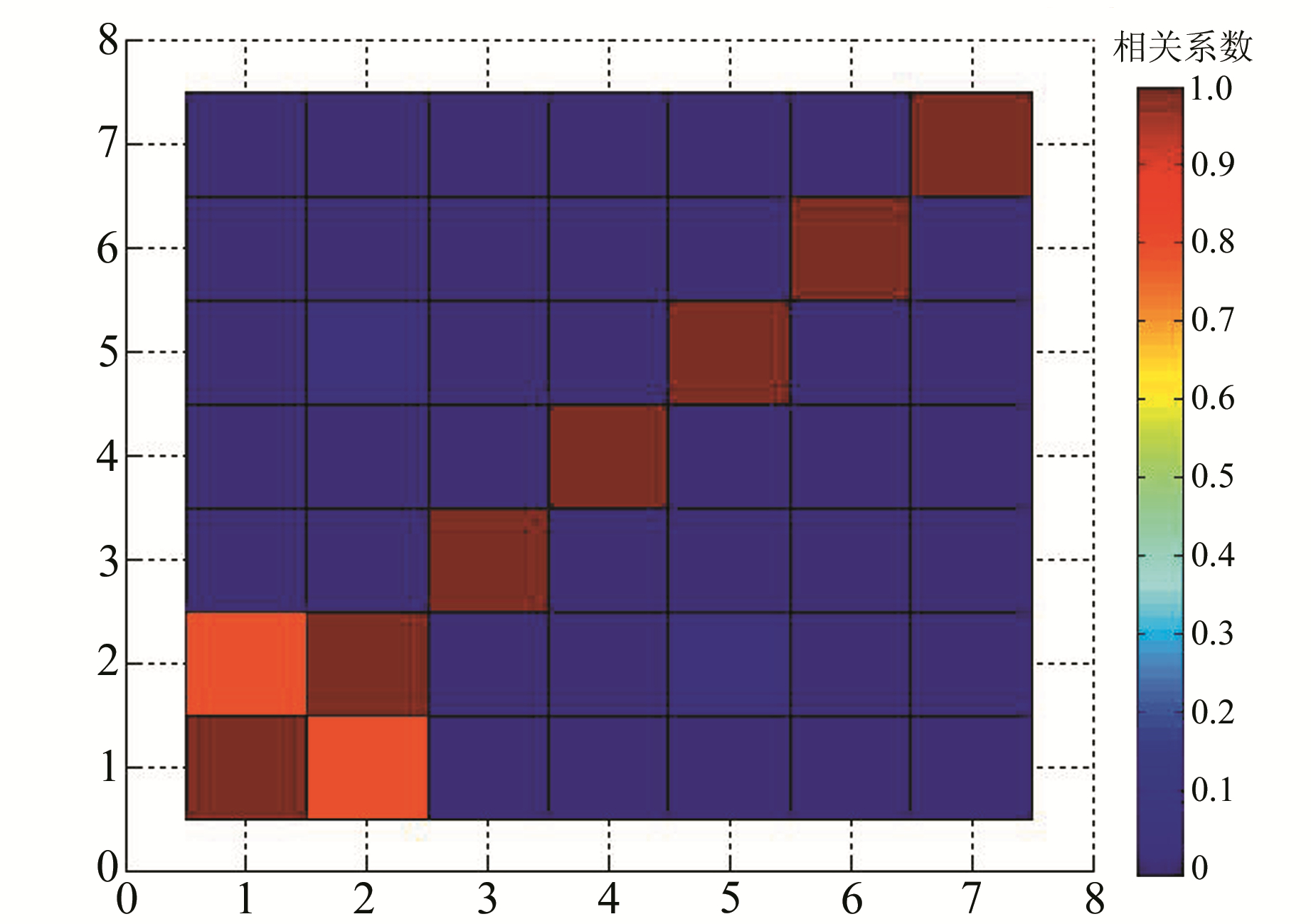图 7 前8阶重构部分的W-correlation Fig. 7 W-correlation of the first 8th-order reconstruction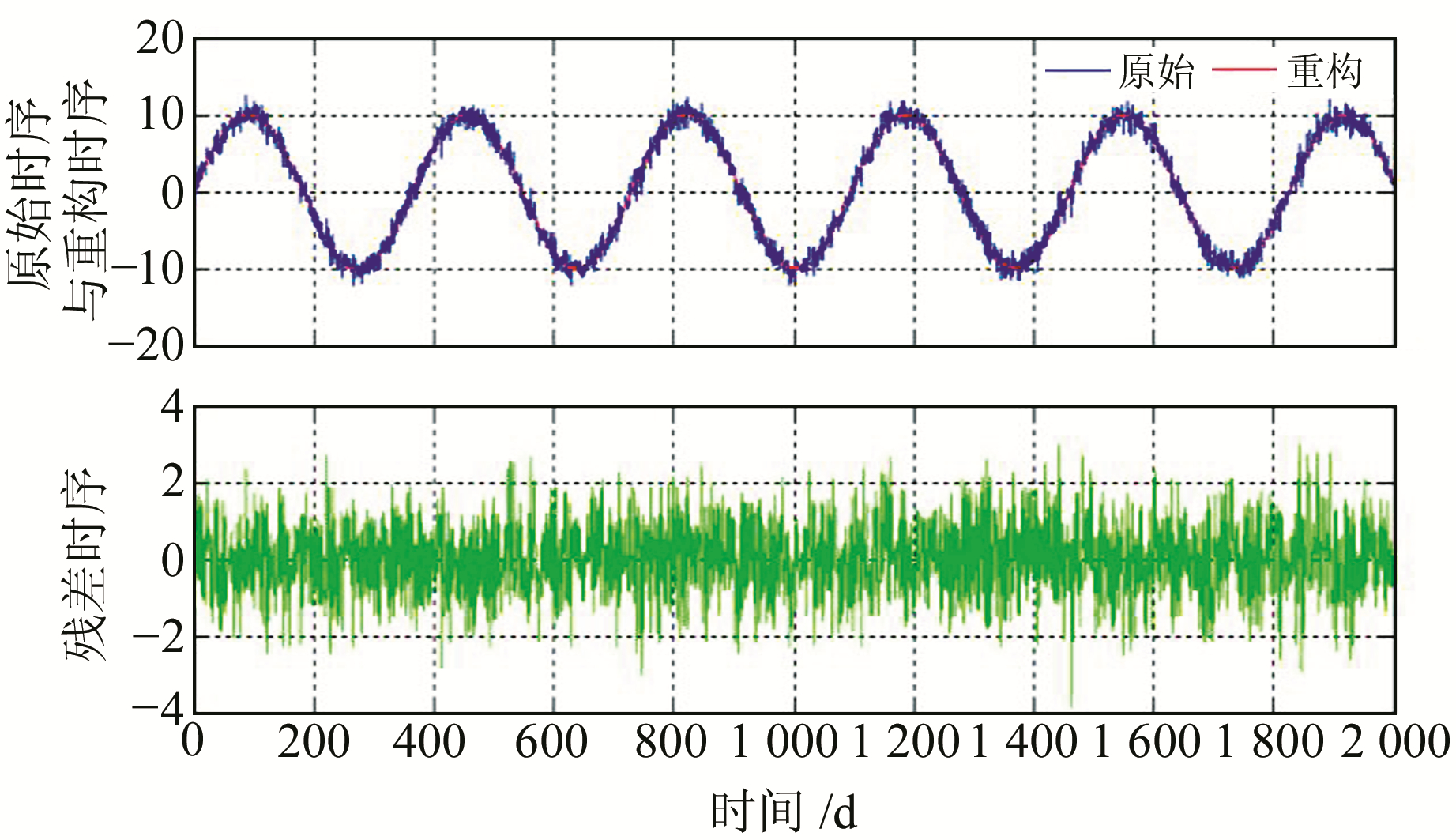图 8 CORS大地高变化模拟时序与重构时序及残差序列 Fig. 8 Simulated and reconstructed and residual time series from CORS geodetic height change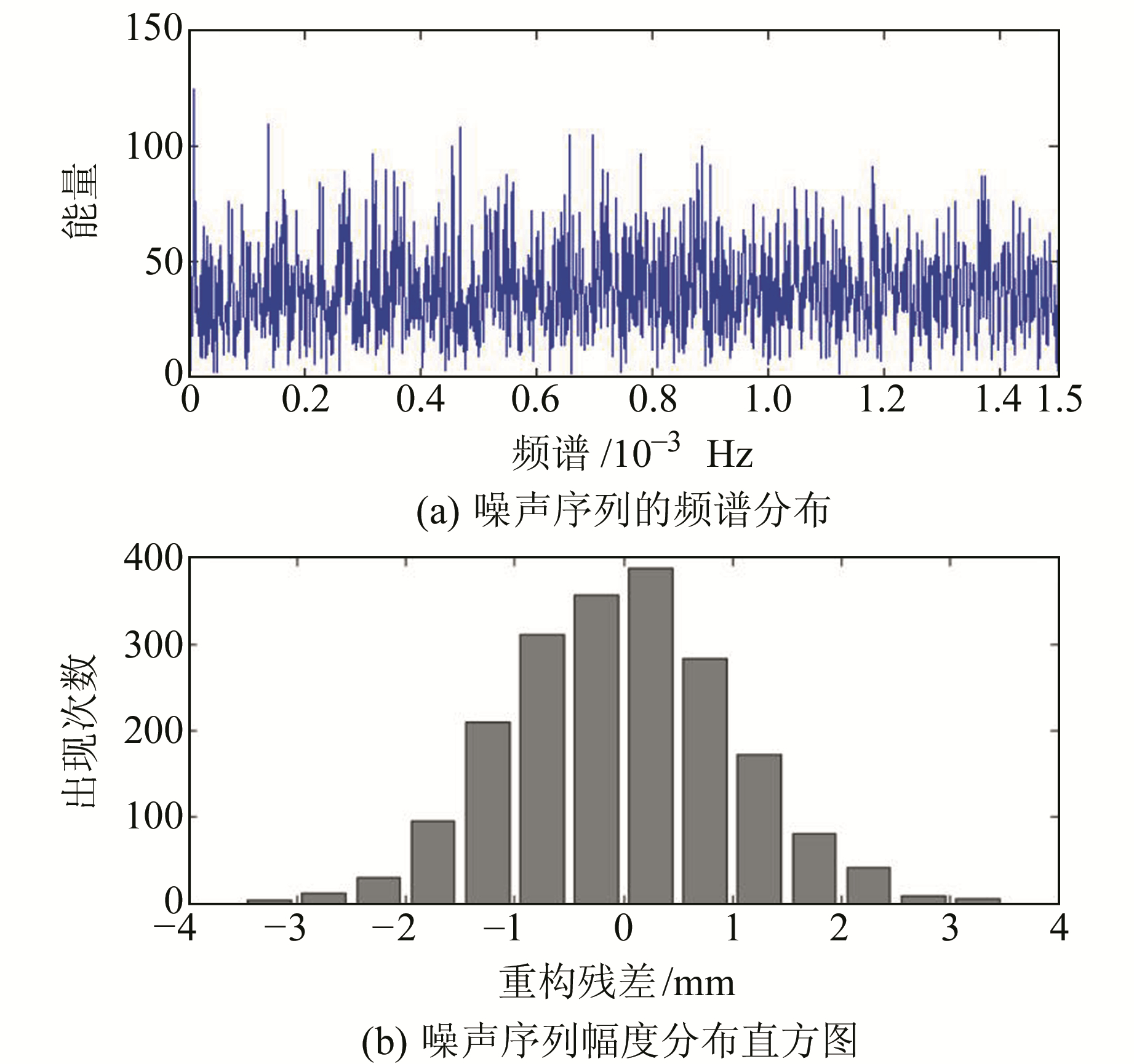图 9 噪声序列的频谱分布与幅度分布直方图 Fig. 9 Spectrum distribution and amplitude distribution histogram of the noise sequence表 1 CORS大地高变化模拟时序及重构时序的均值与标准差 Tab. 1 Mean and standard deviation of simulation and reconstruction timing of geodetic height change of CORS

2.2 CORS大地高时序形变信息提取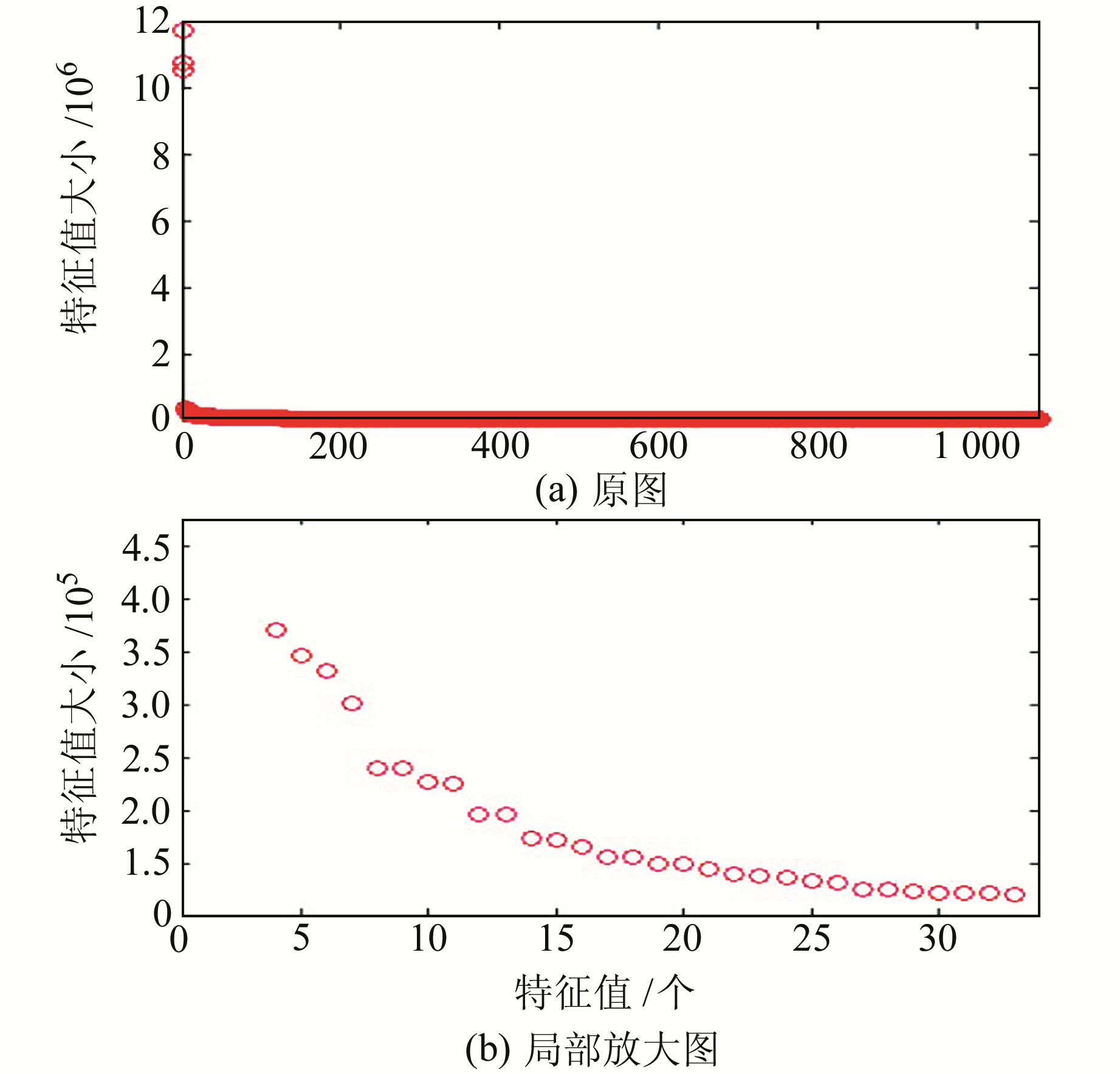图 10 CORS大地高变化实际时序的特征值变化曲线 Fig. 10 Curve of eigenvalues of the actual time series of geodetic height change from CORS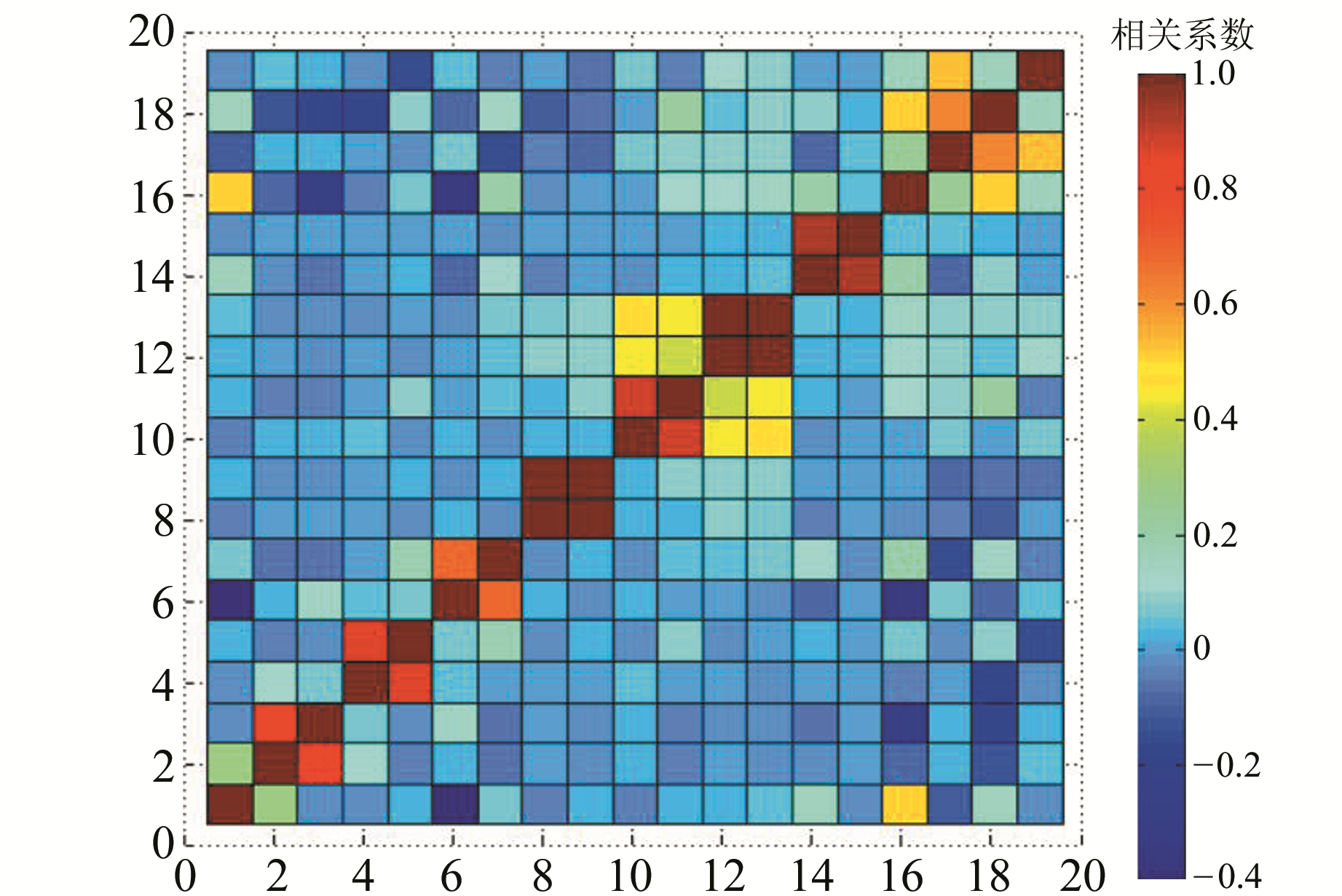图 11 前20阶重构部分的W-correlation Fig. 11 W-correlation of the first 20th-order reconstruction图 12 CORS大地高时序各RC成分及对应的频谱分布 Fig. 12 RC components from geodetic height time series of CORS and corresponding spectral distribution表 2 SSA方法得到的GSLX站周期统计 Tab. 2 Cycle statistics from GSLX station obtained by SSA method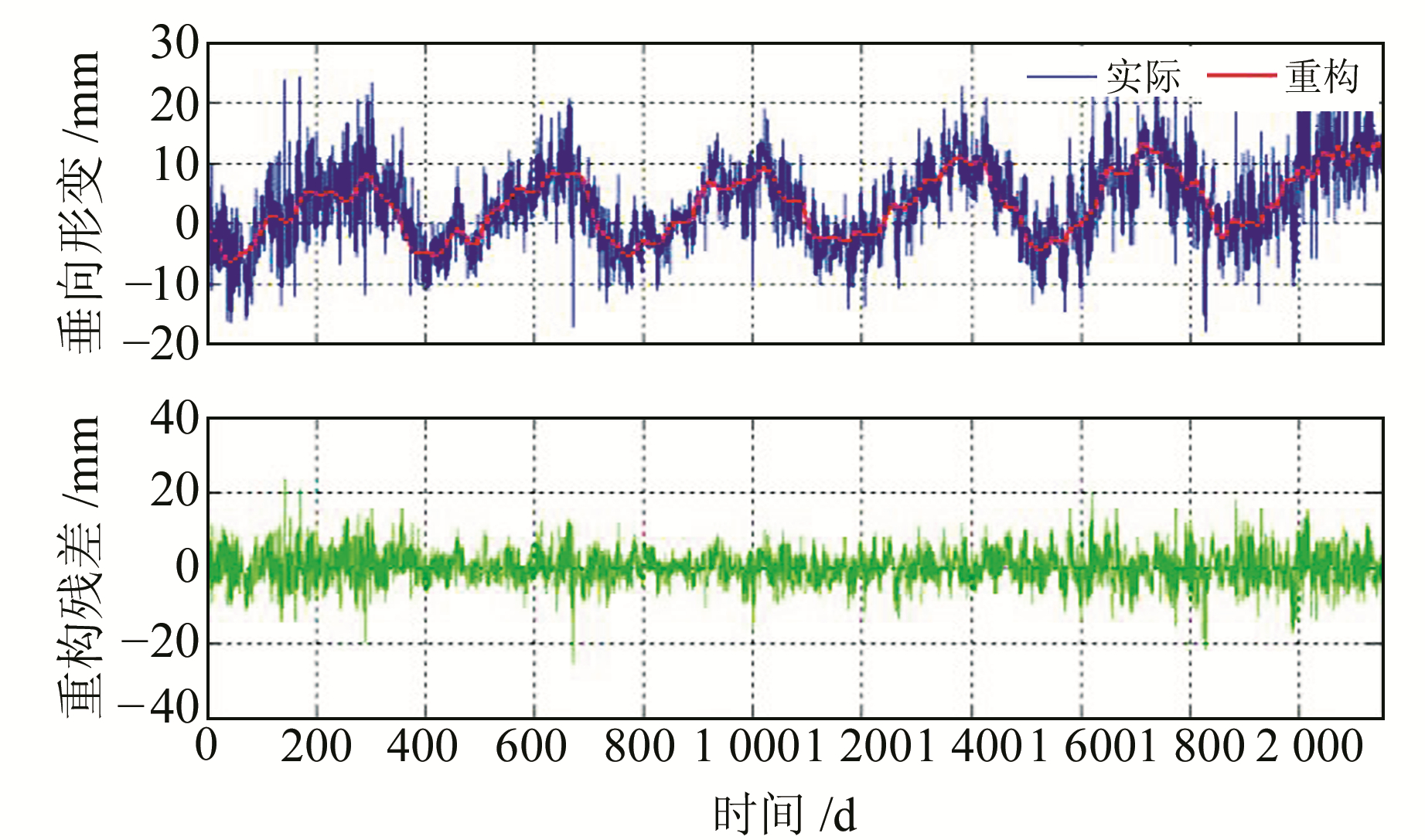图 13 GSLX站大地高变化实际时序与重构时序及残差序列 Fig. 13 Actual and reconstructed and residual time series from GSLX station geodetic height change表 3 CORS大地高时序形变信息提取结果相关统计 Tab. 3 Statistics on extraction results of deformation information on geodetic height timing from CORS

3 结语

  张勇, 吕达仁. 一种基于海面背景的WindSat极化通道替代定标方法研究[J]. 遥感技术与应用, 2014, 29(5): 727-734 (Zhang Yong, Lü Daren. Vicarious Calibration of WindSat Polarimetric Channels Based Sea Surface Background[J]. Remote Sensing Technology and Application, 2014, 29(5): 727-734) (0)  Vautard R, Yiou P, Ghil M. Singular-Spectrum Analysis: A Toolkit for Short, Noisy Chaotic Signals[J]. Physica D:Nonlinear Phenomena, 1992, 58(1-4): 95-126 DOI:10.1016/0167-2789(92)90103-T (0)  Shen Y, Guo J Y, Liu X, et al. Long-Term Prediction of Polar Motion Using a Combined SSA and ARMA Model[J]. Journal of Geodesy, 2018, 92(3): 333-343 DOI:10.1007/s00190-017-1065-3 (0)  王解先, 连丽珍, 沈云中. 奇异谱分析在GPS站坐标监测序列分析中的应用[J]. 同济大学学报:自然科学版, 2013, 41(2): 282-288 (Wang Jiexian, Lian Lizhen, Shen Yunzhong. Application of Singular Spectral Analysis to GPS Station Coordinate Monitoring Series[J]. Journal of Tongji University:Natural Science, 2013, 41(2): 282-288) (0)  Nikolaidis R. Observation of Geodetic and Seismic Deformation with the Gobal Positioning System[D]. San Diego: University of California, 2002 http://www.mendeley.com/catalog/observation-geodetic-seismic-deformation-global-positioning-system/ (0)  李敬唐. 奇异谱分析滤波法及其在GPS多路径去噪中的应用[J]. 测绘地理信息, 2017, 42(3): 10-13 (Li Jingtang. Singular Spectrum Analysis and Its Application in GPS Multipath Filtering[J]. Journal of Geomatics, 2017, 42(3): 10-13) (0)  毛鹏宇, 陈义, 孟鑫. 基于奇异谱分析的地基GPS反演可降水量可行性分析[J]. 大地测量与地球动力学, 2017, 37(9): 933-936 (Mao Pengyu, Chen Yi, Meng Xin. Feasibility Analysis of Precipitable Water Vapor Retrieval with Ground-Based GPS Based on Singular Spectrum Analysis[J]. Journal of Geodesy and Geodynamics, 2017, 37(9): 933-936) (0)  Petit G, Luzum B. IERS Conventions 2010[Z].2010 (0)  Hassani H, Mahmoudvand R, Zokaei M. Separability and Window Length in Singular Spectrum Analysis[J]. Comptes Rendus Mathematique, 2011, 349(17-18): 987-990 DOI:10.1016/j.crma.2011.07.012 (0)  Hsieh W W. Nonlinear Multivariate and Time Series Analysis by Neural Network Methods[J]. Reviews of Geophysics, 2004, 42(1): 1 003-1 027 (0)
Deformation Signal Extraction of Geodetic Height Change Time Series of CORS Based on Singular Spectrum Analysis
LI Wanqiu1,2WANG Wei1ZHANG Chuanyin1     ZHONG Yulong3     YIN Cai4     ZHU Yu5
1. Chinese Academy of Surveying and Mapping, 28 West-Lianhuachi Road, Beijing 100830, China;
2. College of Geomatics, Shandong University of Science and Technology, 579 Qianwangang Road, Qingdao 266590, China;
3. Faculty of Information Engineering, China University of Geosciences, 388 Lumo Road, Wuhan 430074, China;
4. Qingdao Geotechnical Investigation and Surveying Research Institute, 189 Shandong Road, Qingdao 266032, China;
5. Institute of International Rivers and Eco-Security, Yunnan University, Yunxue Road, Kunming 650500, China
Abstract: In order to enhance the signal-to-noise ratio (SNR) of signal extraction, the singular spectrum analysis (SSA) method is used to decompose and reconstruct the geodesic height time series, using the simulation experiment to determine the principle of selecting the embedding dimension and the number of truncated principal components. Furthermore, the SSA method is applied to the extraction of deformation information of CORS. By FFT analysis, we find that the geodetic height time series mainly consists of decomposed seasonal variation components, and the standard deviation of time series after reconstruction is significantly reduced. Our research shows that the SSA method can effectively improve the geodetic height time series SNR of CORS and play a role in noise reduction and smoothing. The research results have certain significance for the expression of vertical deformation of the crust and the expansion of SSA applications.
Key words: CORS; geodetic height time series; signal extraction; SSA; SNR